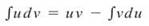﻿ ﻿QUICK REFERENCE GUIDE - Master AP Calculus AB & BC

## Master AP Calculus AB & BC

QUICK REFERENCE GUIDE

These pages contain just about all the formulas you need to know by heart before you can take the AP Test. It does not contain all the theorems and techniques you need to know. An asterisk (*) indicates a Calculus BC-only formula.

LimitsDerivatives

Power Rule:Product Rule:Quotient Rule:Chain Rule:*Parametric derivatives:Derivative Applications

Mean Value Theorem:s(t) is the position function; s’(t) = v(t), the velocity function; v’(t) = a(t), the acceleration function

Projectile position equation:Integration

Power Rule for Integrals:Trapezoidal Rule:Fundamental Theorem (Part 1):if F is the antiderivative of f(x)

Fundamental Theorem (Part 2):Average Value:*Integration by Parts:Integration Applications

Disk Method:Washer Method:Shell Method:*Arc Length:*Polar Area:Differential Equations

Exponential growth/decay:*Logistic growth:*Sequences and Series

Sum of a geometric series:Ratio Test:Limit Comparison Test:Taylor series for f(x) centered at x = c:﻿